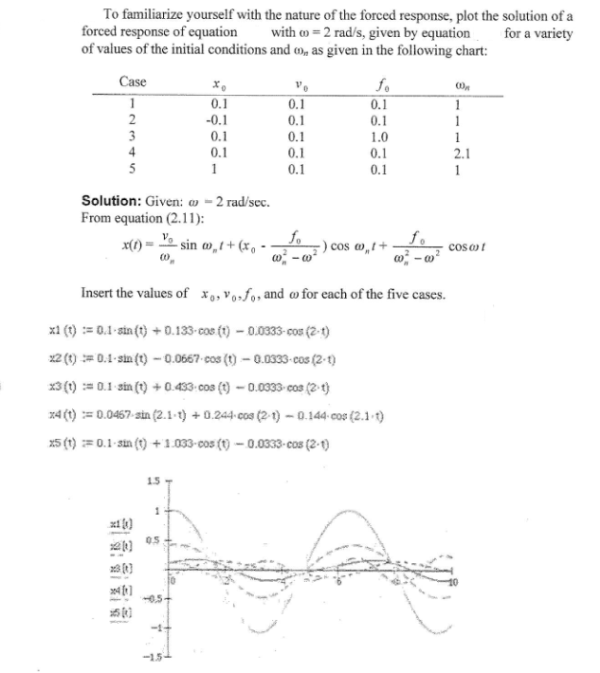To familiarize yourself with the nature of the forced response, plot the solution of a forced response of equation with w=2 rad/s, given by equation for a variety of values of the initial conditions and wn as given in the following chart.To familiarize yourself with the nature of the forced response, plot the solution of a forced response of equation with w=2 rad/s, given by equation for a variety of values of the initial conditions and wn as given in the following chart.

Vibrations Page 1 vibrations vibrations vibrations vibrations vibrations vibrations vibrations Vibrations Page 2